Courses

# Test: One-Dimensional Steady-State Conduction - 2

## 10 Questions MCQ Test Topicwise Question Bank for Mechanical Engineering | Test: One-Dimensional Steady-State Conduction - 2

Description
This mock test of Test: One-Dimensional Steady-State Conduction - 2 for Chemical Engineering helps you for every Chemical Engineering entrance exam. This contains 10 Multiple Choice Questions for Chemical Engineering Test: One-Dimensional Steady-State Conduction - 2 (mcq) to study with solutions a complete question bank. The solved questions answers in this Test: One-Dimensional Steady-State Conduction - 2 quiz give you a good mix of easy questions and tough questions. Chemical Engineering students definitely take this Test: One-Dimensional Steady-State Conduction - 2 exercise for a better result in the exam. You can find other Test: One-Dimensional Steady-State Conduction - 2 extra questions, long questions & short questions for Chemical Engineering on EduRev as well by searching above.
QUESTION: 1

Solution:
QUESTION: 2

### The temperature distribution for a hollow cylinder for steady state heat flow and constant value of thermal conductivity is

Solution:

Since heat transfer in hollow cylinder is given by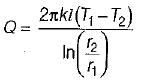Hence heat transfer profile is logarithmic.

QUESTION: 3

### The temperature distribution for a sphere for steady state heat flow and constant value of thermal conductivity is

Solution: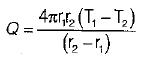Hence profile will be hyperbolic.

QUESTION: 4

The heat transfer in the hollow cylinder is taking place by conduction and in this case logarithmic mean temperature difference Alm is given by

Solution:

Heat transfer by conduction through hollow cylinder is given by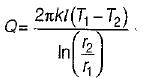Also,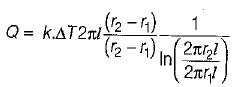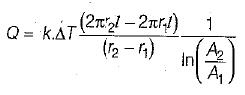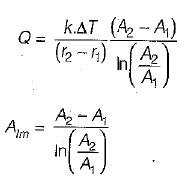QUESTION: 5

What will be geometric radius of heat transfer for a hollow sphere of inner and outer radii r1 and r2?

Solution:

Heat transfer by conduction through sphere is given by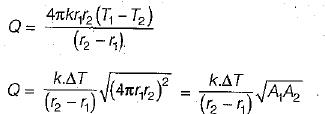Geometric mean Area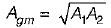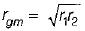QUESTION: 6

Thermal diffusivity is given by

Solution:

Thermal diffusivity is the ratio of thermal conductivity and thermal diffusivity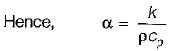QUESTION: 7

A composite slab has two layers of different materials with thermal conductivity k1 and k2. In each layer had the same thickness, the, equivalent thermal conductivity of the slab would be

Solution: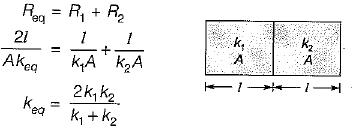QUESTION: 8

A steam pipe is covered with two layers of insulating materials, with the better insulating material forming the outer part. If the two layers are interchanged, the heat conducted

Solution: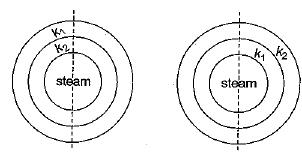k1 < k2 [k1 better insulator]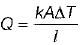Q ∝ k. This means that the material having better insulating materia! should be inside to minimize the heat flux hence if better insulating materia! is placed inside, it will reduce the heat transfer.

QUESTION: 9

In a long cylindrical rod of radius R and a surface heat flux of q0 the uniform generation rate is

Solution: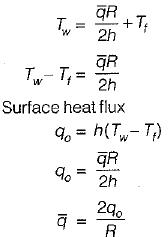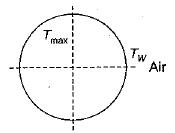QUESTION: 10

The critical thickness of insulation for cylinder is given by

Solution: